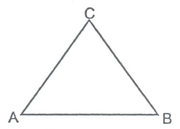# Introduction

## TRIANGLE

A plane figure bounded by three lines in a plane is called a triangle. Every triangle have three sides and three angles. If ABC is any triangle then AB, BC & CA are three sides and ∠A, ∠B and ∠C are three angles.We have studied about triangles and their different properties in our previous classes. We know that a closed geometrical figure formed by three intersecting lines is called a triangle. Tri means three, So, a triangle has three sides, three angles and three vertices.

e.g. In Fig., ABC is a triangle i.e. in ΔABC, AB, BC and AC are three sides, ∠A, ∠B and ∠C are three angles and A, B and C are its three vertices.